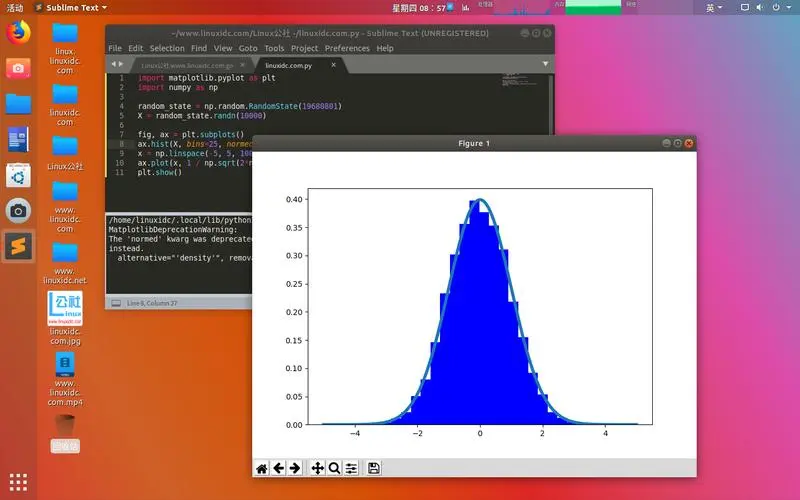# JavaScript实现伪随机正态分布

2023-09-24 6 0

• 💂 个人网站:【海拥】【游戏大全】【神级源码资源网】
• 🤟 前端学习课程：👉【28个案例趣学前端】【400个JS面试题】
• 💅 寻找学习交流、摸鱼划水的小伙伴，请点击【摸鱼学习交流群】

#### 目录

• 前言
• 什么是正态分布？
• 伪随机数生成算法
• 实现伪随机正态分布
• 1 Box-Muller转换方法
• 2 使用概率密度函数逆变换法
• 总结### 实现伪随机正态分布

#### 1 Box-Muller转换方法

Box-Muller转换方法是一种常用的生成正态分布的方法。它利用了两个独立且均匀分布的随机数，并将其转换为服从正态分布的随机数。

#### 2 使用概率密度函数逆变换法

``````// 使用Box-Muller方法生成伪随机正态分布
function generateNormalDistribution(mean, stdDev) {let u = 0, v = 0;while (u === 0) u = Math.random(); // 生成(0,1)区间的随机数while (v === 0) v = Math.random(); // 生成(0,1)区间的随机数let z0 = Math.sqrt(-2.0 * Math.log(u)) * Math.cos(2.0 * Math.PI * v); // 第一个正态分布随机数let z1 = Math.sqrt(-2.0 * Math.log(u)) * Math.sin(2.0 * Math.PI * v); // 第二个正态分布随机数return z0 * stdDev + mean; // 转换为指定均值和标准差的正态分布随机数
}// 示例使用
let mean = 0; // 均值
let stdDev = 1; // 标准差
let randomValue = generateNormalDistribution(mean, stdDev);
console.log(randomValue);
``````